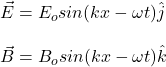## To understand the formula representing a traveling electromagnetic wave. Light, radiant heat (infrared radiation), X rays, and

Question

To understand the formula representing a traveling electromagnetic wave.

Light, radiant heat (infrared radiation), X rays, and radio waves are all examples of traveling electromagnetic waves. Electromagnetic waves comprise combinations of electric and magnetic fields that are mutually compatible in the sense that the changes in one generate the other.

The simplest form of a traveling electromagnetic wave is a plane wave. For a wave traveling in the x direction whose electric field is in the y direction, the electric and magnetic fields are given by

E? =E0sin(kx??t)j^,
B? =B0sin(kx??t)k^.

This wave is linearly polarized in the y direction.

1.a. In these formulas, it is useful to understand which variables are parameters that specify the nature of the wave. The variables E0 and B0are the __________ of the electric and magnetic fields.

Choose the best answer to fill in the blank.

1. maxima
2. amplitudes
3. wavelengths
4. velocities

b. The variable ? is called the __________ of the wave.

Choose the best answer to fill in the blank.

1. velocity

2. angular frequency

3. wavelength

c. The variable k is called the __________ of the wave.

Choose the best answer to fill in the blank.

1. wavenumber

2. wavelength

3. velocity

4. frequency

d. What is the mathematical expression for the electric field at the point x=0,y=0,z at time t?

1. E=E0sin(??t)j^

2. E =E0sin(??t)k^

3. E =0

4. E =E0sin(kz??t)i^

5. E =E0sin(kz??t)j^

e. For a given wave, what are the physical variables to which the wave responds?

1. x only

2. t only

3. k only

4. ? only

5. x and t

6. x and k

7. ? and t

8. k and ?

in progress 0
6 months 2021-07-15T02:06:34+00:00 1 Answers 6 views 0

## Answers ( )

Explanation:

We have that both electric field and magnetic field are given by:I complete with bold words the answers:

_ _ _ _ _ _ _ _ _ _ _ _ _ _ _ _

a. In these formulas, it is useful to understand which variables are parameters that specify the nature of the wave. The variables E0 and B0are the magnitudes of the electric and magnetic fields.

2. amplitudes

b. The variable w is called the angular frequency of the wave.

2. angular frequency

c. The variable k is called the wavenumber of the wave.

1. wavenumber

c.

1. E =E0sin(wt)k^

d.

6. x and t

hope this helps!!